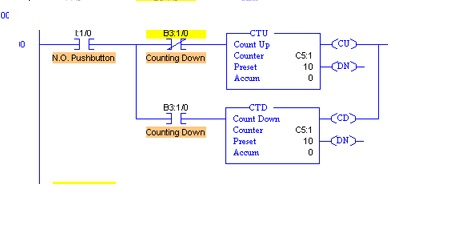# COUNTERS in PLC Programming### Introduction

There are two basic counter types: count-up and count-down. When the input to a count-up counter goes true the accumulator value will increase by 1 (no matter how long the input is true.) If the accumulator value reaches the preset value the counter DN bit will be set. A count-down counter will decrease the accumulator value until the preset value is
reached.In this session we are going to seperatley look into the 2 counters in PLC programming.

An Allen Bradley count-up (CTU) instruction is shown in Figure. The instruction requires memory in the PLC to store values and status, in this case is example. The preset value is 4 and the value in the accumulator is 2. If the input A were to go from false to true the value in the accumulator would increase to 3. If A were to go off, then on again the accumulator value would increase to 4, and the DN bit would go on. The count can continue above the preset value. If input B becomes true the value in the counter accumulator will become zero.Counters are used in applications that require items or products to be counted.

• Counter instructions contain three words. The bits and words of counter instructions have addresses that can be used in the program of a PLC.
• All counter instructions consist of three words: word 0, word 1, and word 2. Each word contains 16 bits.Word 0 contains five bits that are available for use in a PLC program:
• bit 15—Count up (CU) enable
• bit 14—Countdown (CD) enable
• bit 13—done (DN)
• bit 12—overflow (OV)
• bit 11—underflow (UN)### Counter in PLC: Count up (CTU) instruction

• A count up instruction (CTU) is a PLC programming instruction used to count the number of operations or products produced by a system.
• A CTU instruction counts every false to true transition from the logic that precedes the instruction in a rung.
• The cumulative value of a CTU instruction increases by one for each transition from false to true.
• A CTU instruction will allow a counter to continue counting beyond the preset value. An identified RES instruction is required to reset a CTU instruction.### Counters in PLC : Countdown(CTD) instruction

• When used together, the CTD and CTU instructions have the same address, similar to the OTL and OTU instructions.
• The CTU and CTD instructions share the same status bits, presets and accumulated values. However, each counting instruction has its own logic.
• A CTD instruction counts down every false-to-true transition from the logic that precedes the instruction in a rung.
• The cumulative value of a CTD instruction decreases by one for each false to true transition of the logic that precedes a CTD instruction in a rung. The other way
• A CTU instruction causes the accumulated value of a CTD instruction to increase. A single RES instruction of identical address is required to reset the CTD and CTU instructions. Next, the image shows the CTD instruction and the related bit.### Siemens Counter program is given below

When Pump_Run is toggled on, Delay_Tmr begins to time and Delay_Tmr.DN turns on 500 msec after Pump_Run (Delay_Tmr.EN) turns on. With the counter, when Part_Present is toggled on, Part_Count increments by one. When Part_Present is toggled 1000 times, Part_Count.DN turns on.